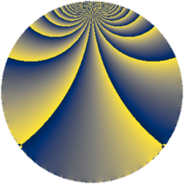Properties

 Label 252.1.zLevel $252$ Weight $1$ Character orbit 252.z Rep. character $\chi_{252}(73,\cdot)$ Character field $\Q(\zeta_{6})$ Dimension $2$ Newform subspaces $1$ Sturm bound $48$ Trace bound $0$

Related objects

Defining parameters

 Level: $$N$$ $$=$$ $$252 = 2^{2} \cdot 3^{2} \cdot 7$$ Weight: $$k$$ $$=$$ $$1$$ Character orbit: $$[\chi]$$ $$=$$ 252.z (of order $$6$$ and degree $$2$$) Character conductor: $$\operatorname{cond}(\chi)$$ $$=$$ $$7$$ Character field: $$\Q(\zeta_{6})$$ Newform subspaces: $$1$$ Sturm bound: $$48$$ Trace bound: $$0$$

Dimensions

The following table gives the dimensions of various subspaces of $$M_{1}(252, [\chi])$$.

Total New Old
Modular forms 26 2 24
Cusp forms 2 2 0
Eisenstein series 24 0 24

The following table gives the dimensions of subspaces with specified projective image type.

$$D_n$$ $$A_4$$ $$S_4$$ $$A_5$$
Dimension 2 0 0 0

Trace form

 $$2 q + q^{7} + O(q^{10})$$ $$2 q + q^{7} - 3 q^{19} - q^{25} - 3 q^{31} - q^{37} + 2 q^{43} - q^{49} + q^{67} + 3 q^{73} + q^{79} + 3 q^{91} + O(q^{100})$$

Decomposition of $$S_{1}^{\mathrm{new}}(252, [\chi])$$ into newform subspaces

Label Dim. $$A$$ Field Image CM RM Traces $q$-expansion
$a_{2}$ $a_{3}$ $a_{5}$ $a_{7}$
252.1.z.a $2$ $0.126$ $$\Q(\sqrt{-3})$$ $D_{6}$ $$\Q(\sqrt{-3})$$ None $$0$$ $$0$$ $$0$$ $$1$$ $$q-\zeta_{6}^{2}q^{7}+(\zeta_{6}+\zeta_{6}^{2})q^{13}+(-1-\zeta_{6}+\cdots)q^{19}+\cdots$$